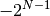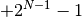# numpy.binary_repr¶

numpy.binary_repr(num, width=None)

Return the binary representation of the input number as a string.

For negative numbers, if width is not given, a minus sign is added to the front. If width is given, the two’s complement of the number is returned, with respect to that width.

In a two’s-complement system negative numbers are represented by the two’s complement of the absolute value. This is the most common method of representing signed integers on computers [R16]. A N-bit two’s-complement system can represent every integer in the rangeto.

Parameters : num : int Only an integer decimal number can be used. width : int, optional The length of the returned string if num is positive, the length of the two’s complement if num is negative. bin : str Binary representation of num or two’s complement of num.

base_repr
Return a string representation of a number in the given base system.

Notes

binary_repr is equivalent to using base_repr with base 2, but about 25x faster.

References

 [R16] (1, 2) Wikipedia, “Two’s complement”, http://en.wikipedia.org/wiki/Two’s_complement

Examples

```>>> np.binary_repr(3)
'11'
>>> np.binary_repr(-3)
'-11'
>>> np.binary_repr(3, width=4)
'0011'
```

The two’s complement is returned when the input number is negative and width is specified:

```>>> np.binary_repr(-3, width=4)
'1101'
```

numpy.unpackbits

Statistics